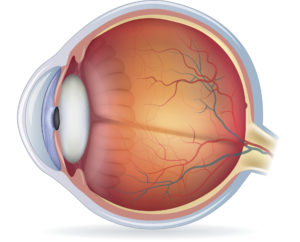Research Review

# Can the AL/CR Ratio Alone Be Used to Determine the Magnitude and/or Progression of Myopia?

Feb. 4, 2020

By Nicole Xiao Liu, MOptm
PhD Candidate, Brien Holden Vision InstituteConventionally, myopia studies use spherical equivalent and axial length to classify refractive status as well as to monitor the progression of the condition. In recent years, however, the axial length/corneal radius of curvature ratio (AL/CR ratio) is proposed as a more robust indicator of the refractive status of the eye due to the fact that it takes into account the relation between axial length and corneal radius. Compared with axial length alone, the AL/CR ratio showed a stronger correlation with spherical equivalent. The ratio itself, when used for myopia classification, is found independent of ethnicity in many studies. Therefore, one might wonder: can the AL/CR ratio alone be used to determine the magnitude and/or progression of myopia?

To answer this question, Jong et al. (2018) conducted a large-scale retrospective study analyzing axial length, corneal radii of curvature and cycloplegic objective refraction in children with either low myopia (n = 732; age 7 to 16 years; myopia sphere −0.50 to −3.50 D; cylinder ≤1.00 D) or high myopia (n = 308; age 7 to 16 years; myopia sphere −6.00 D or worse). The relationship between the AL/CR ratio and the magnitude of myopia (cycloplegic spherical equivalent) was analyzed and compared between low and high myopia groups. Children with low myopia were furthermore divided into two groups: fast progressing group (≥0.75 D myopic shift) and slow-progressing group (<0.75 D myopic shift) based on their annual cycloplegic spherical equivalent changes. Relationships of the AL/CR ratio increase and myopia progression were compared between the two groups.

Interestingly, the authors found that the relationship between AL/CR ratio and spherical equivalent (SE) was nonlinear (quadratic fit, R2=0.84) and age-adjusted slopes for low and high myopia groups were significantly different (P < 0.05), which indicates that the AL/CR ratio can be used to differentiate the types of myopia (high myopia vs. low myopia) but cannot be used as a replacement for SE. In other words, since the correlation between AL/CR ratio and SE varies across different ametropias, the ratio alone cannot reveal the magnitude of myopia.

In terms of using the AL/CR ratio to monitor myopia progression, the authors suggested that the ratio is of little value in determining the progression of myopia. Compared with axial length, the AL/CR ratio explained less variance in annual SE changes (R2=0.52 versus R2=0.45). The correlations were further weakened after adjusting for age in both fast and slow progressing groups (R2=0.38 and R2=0.19, respectively).

To conclude, as the first large-scale retrospective study investigating whether the AL/CR ratio alone can be employed to determine the magnitude and/or annual progression of myopia, this study has rejected such a proposal with solid evidence.

The Relationship between Progression in Axial Length/Corneal Radius of Curvature Ratio and Spherical Equivalent Refractive Error in Myopia.

Jong M, Sankaridurg P, Naduvilath TJ, Li W, He M.

Abstract

SIGNIFICANCE: This study demonstrates that mean axial length/corneal radius of curvature ratio (AL/CR) can be used to detect low and high myopia but cannot clinically monitor myopia progression because the relationship between AL/CR and progression in myopia is different between low and high myopia.

PURPOSE: The purpose of this study was to investigate the relationship of AL/CR with magnitude and progression of myopia.

METHODS: Retrospective analysis was conducted comparing the right eyes of those with high myopia (n = 308; age, 7 to 16 years; myopia sphere, −6.00 diopters or worse) with those with low myopia (n = 732; age, 7 to 16 years; myopia sphere, between −0.50 and −3.50 diopters; cylinder, ≤1.00 diopters). Baseline axial length, corneal radii of curvature, and cycloplegic objective refraction were analyzed. Myopia progression in the low-myopia group at 6- and 12-month follow-up was measured, and the differences in slopes of AL/CR were compared for slow (<0.75 diopters) and fast progressing (≥0.75 diopters).

RESULTS: Mean AL/CR values were significantly different (P < .001) between high myopia (3.46 ± 0.10) and low myopia (3.16 ± 0.07). In high and low myopia, slopes of axial length versus corneal curvature radius were not significantly different (P > .05), and slopes of AL/CR versus spherical equivalent were significantly different after adjusting for spherical equivalent and age (P < .05). Slopes of AL/CR progression and spherical equivalent progression were significantly different in low myopia between fast and slow progressing (P < .001), but the relationship between progression in AL/CR and progression in spherical equivalent was not strong.

CONCLUSIONS: The AL/CR can be used to classify different grades of myopia, but it is not useful in determining the magnitude of myopia or monitoring progression because AL/CR is not linearly related to spherical equivalent and because progression in AL/CR is not strongly related to spherical equivalent progression.

Jong, M., Sankaridurg, P., Naduvilath, T. J., Li, W., & He, M. (2018). The relationship between progression in axial length/corneal radius of curvature ratio and spherical equivalent refractive error in myopia. Optometry and Vision Science, 95(10), 921-929.

DOI: 10.1097/OPX.0000000000001281

To Top